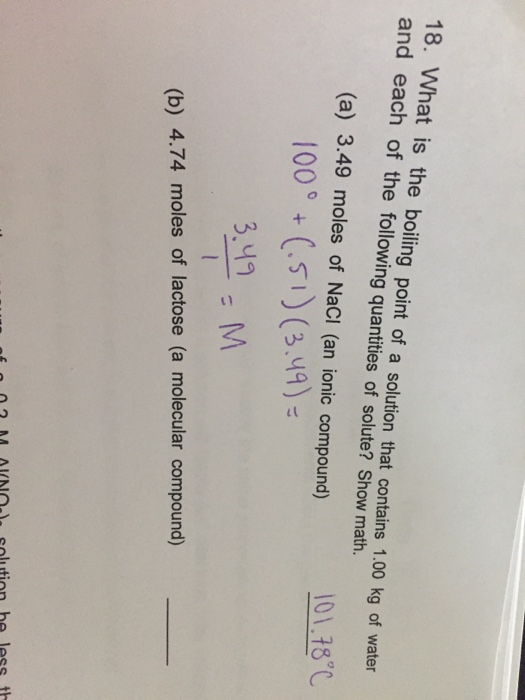# Question & Answer: What is the boiling point of a solution that contains 1.00 kg of water and each of the following…..

I think I might’ve done this problem wrong, help please??What is the boiling point of a solution that contains 1.00 kg of water and each of the following quantities of solute? Show math. (a) 3.49 moles of NaCl (an ionic compound) _____ (b) 4.74 moles of lactose (a molecular compound) _____

a) NaCl is ionic compound it dissociates to ions so i = 2

molality = moles / mass of solvent = 3.49 / 1 = 3.49 m

Don't use plagiarized sources. Get Your Custom Essay on
Question & Answer: What is the boiling point of a solution that contains 1.00 kg of water and each of the following…..
GET AN ESSAY WRITTEN FOR YOU FROM AS LOW AS \$13/PAGE

Tb = i x Kb x m

Tb = 2 x 0.512 x 3.49

Tb = 3.57

boiling point of solution = 100 + 3.57 = 103.570C

b) as lactate is molecular compound i = 1

molality = 4.74 / 1 = 4.74

Tb = 0.512 x 4.74

Tb = 2.43

boiling point of solution = 100 + 2.43 = 102.430C